1. /
2. CBSE
3. /
4. Class 06
5. /
6. Mathematics
7. /
8. NCERT Solutions for Class...

# NCERT Solutions for Class 6 Maths Exercise 14.2

NCERT solutions for Maths Practical geometry## NCERT Solutions for Class 6 Maths Practical geometry

###### Question 1.Draw a line segment of length 7.3 cm, using a ruler.

(i) Place the zero mark of the ruler at a point A.

(ii) Mark a point B at a distance of 7.3 cm from A.

(iii) Join AB.

{tex}\overline {{\text{AB}}} {/tex} is the required line segment of length 7.3 cm.

NCERT Solutions for Class 6 Maths Exercise 14.2

###### Question 2.Construct a line segment of length 5.6 cm using ruler and compasses.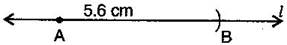(i) Draw a line {tex}’l’.{/tex} Mark a point A on this line.

(ii) Place the compasses pointer on zero mark of the ruler. Open it to place the pencil point up to 5.6 cm mark.

(iii) Without changing the opening of the compasses. Place the pointer on A and cut an arc {tex}’l'{/tex} at B.

{tex}\overline {{\text{AB}}} {/tex} is the required line segment of length 5.6 cm.

NCERT Solutions for Class 6 Maths Exercise 14.2

###### Question 3.Construct {tex}\overline {{\text{AB}}} {/tex} of length 7.8 cm. From this cut off {tex}\overline {{\text{AC}}} {/tex} of length 4.7 cm. Measure {tex}\overline {{\text{BC}}} .{/tex}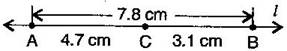(i) Place the zero mark of the ruler at A.

(ii) Mark a point B at a distance 7.8 cm from A.

(iii) Again, mark a point C at a distance 4.7 from A.

By measuring {tex}\overline {{\text{BC}}} {/tex}, we find that BC = 3.1 cm

NCERT Solutions for Class 6 Maths Exercise 14.2

###### Question 4.Given {tex}\overline {{\text{AB}}} {/tex} of length 3.9 cm, construct {tex}\overline {{\text{PQ}}} {/tex} such that the length {tex}\overline {{\text{PQ}}} {/tex} is twice that of {tex}\overline {{\text{AB}}} {/tex}. Verify by measurement.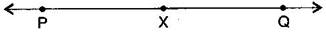(Hint: Construct {tex}\overline {{\text{PX}}} {/tex} such that length of {tex}\overline {{\text{PX}}} {/tex} = length of {tex}\overline {{\text{AB}}} {/tex}; then cut off {tex}\overline {{\text{XQ}}} {/tex} such that {tex}\overline {{\text{XQ}}} {/tex} also has the length of {tex}\overline {{\text{AB}}} {/tex}.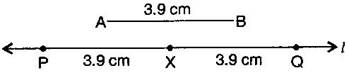(i) Draw a line {tex}’l’.{/tex}

(ii) Construct {tex}\overline {{\text{PX}}} {/tex} such that length of {tex}\overline {{\text{PX}}} {/tex} = length of {tex}\overline {{\text{AB}}} {/tex}

(iii) Then cut of {tex}\overline {{\text{XQ}}} {/tex} such that {tex}\overline {{\text{XQ}}} {/tex} also has the length of {tex}\overline {{\text{AB}}} .{/tex}

(iv) Thus the length of {tex}\overline {{\text{PX}}} {/tex} and the length of {tex}\overline {{\text{XQ}}} {/tex} added together make twice the length of {tex}\overline {{\text{AB}}} .{/tex}

Verification:

By measurement we find that PQ = 7.8 cm

= 3.9 cm + 3.9 cm = {tex}\overline {{\text{AB}}} {/tex} + {tex}\overline {{\text{AB}}} {/tex} = 2 x {tex}\overline {{\text{AB}}} {/tex}

NCERT Solutions for Class 6 Maths Exercise 14.2

###### Question 5.Given {tex}\overline {{\text{AB}}} {/tex} of length 7.3 cm and {tex}\overline {{\text{CD}}} {/tex} of length 3.4 cm, construct a line segment {tex}\overline {{\text{XY}}} {/tex} such that the length of {tex}\overline {{\text{XY}}} {/tex} is equal to the difference between the lengths of {tex}\overline {{\text{AB}}} {/tex} and {tex}\overline {{\text{CD}}} .{/tex} Verify by measurement.

(i) Draw a line {tex}’l'{/tex} and take a point X on it.

(ii) Construct {tex}\overline {{\text{XZ}}} {/tex} such that length {tex}\overline {{\text{XZ}}} {/tex} = length of {tex}\overline {{\text{AB}}} {/tex} = 7.3 cm

(iii) Then cut off {tex}\overline {{\text{ZY}}} {/tex} = length of {tex}\overline {{\text{CD}}} {/tex} = 3.4 cm

(iv) Thus the length of {tex}\overline {{\text{XY}}} {/tex} = length of {tex}\overline {{\text{AB}}} {/tex} – length of {tex}\overline {{\text{CD}}} {/tex}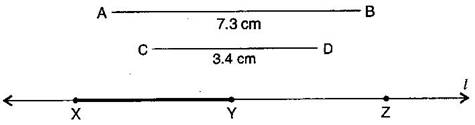Verification:

By measurement we find that length of {tex}\overline {{\text{XY}}} {/tex} = 3.9 cm

= 73. Cm – 3.4 cm = {tex}\overline {{\text{AB}}} – \overline {{\text{CD}}} {/tex}

## NCERT Solutions for Class 6 Maths Exercise 14.2

NCERT Solutions Class 6 Maths PDF (Download) Free from myCBSEguide app and myCBSEguide website. Ncert solution class 6 Maths includes text book solutions from Class 6 Maths Book . NCERT Solutions for CBSE Class 6 Maths have total 14 chapters. 6 Maths NCERT Solutions in PDF for free Download on our website. Ncert Maths class 6 solutions PDF and Maths ncert class 6 PDF solutions with latest modifications and as per the latest CBSE syllabus are only available in myCBSEguide.

## CBSE app for Students

To download NCERT Solutions for Class 6 Maths, Social Science Computer Science, Home Science, Hindi English, Maths Science do check myCBSEguide app or website. myCBSEguide provides sample papers with solution, test papers for chapter-wise practice, NCERT solutions, NCERT Exemplar solutions, quick revision notes for ready reference, CBSE guess papers and CBSE important question papers. Sample Paper all are made available through the best app for CBSE students and myCBSEguide website.### Test Generator

Create question paper PDF and online tests with your own name & logo in minutes.### myCBSEguide

Question Bank, Mock Tests, Exam Papers, NCERT Solutions, Sample Papers, Notes

### 4 thoughts on “NCERT Solutions for Class 6 Maths Exercise 14.2”

1. It’s a useful guide solutions for all of us studying at CBSE board . Thanks a lot for guiding me. your faithful
Viewer.
Miss- Riyanshi Sarkar

2. Awesome

3. It is really very helpful for students. All solutions are well explained. Thanks for guiding us.
Viewer
Neelam Pundhir.

4. Thank you!